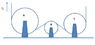# Finding acceleration given normal component [Dynamics]

• tubbis9001

## Homework Statement

[/B]
A belt moves over three pulleys at a constant speed v0 [ft/s]. Knowing that the normal component of the acceleration of the portion of the belt in contact with pulley B is aB,n=90 [ft/s2 ]. Determine:
(a) speed of the belt, v0 [ft/s], and
(b) normal component of the acceleration of the portion of the belt in contact with pulley A, aA,n [ft/s2 ].

Radii of pulleys are: rA =3 [in], rB =1 [in], rC=2.5 [in].

## Homework Equations

an = ||v x a|| / ||v||

## The Attempt at a Solution

[/B]
I believe the first step would be to find the acceleration of the belt given the normal component. Since the belt is moving at a constant speed, both the tangential component and the acceleration would be 0 ft/s2.

After that, we should put the numbers we have into the equation and get:

90 ft/s2 = ||v x 0 ft/s2|| / ||v||

..and solve for velocity. However I'm unsure how to do this since none of the numbers I'm given are in component vector form.

For part B, I would assume it is just aA,n divided by 3 since the radius of A is 3 times larger than B?

#### Attachments

•HW2 diagram.png
6.5 KB · Views: 441
This is not a correct assessment. You are right in saying that the tangential component of acceleration is zero, but all that means is that the normal component of the acceleration vector is the same as the overall acceleration vector.

You have the material particles that comprise the belt traveling in a circular path around the roll. What is the equation for acceleration when a particle travels in a circular path?

Chet